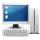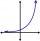# Geometric sequence

In the geometric sequence is a4 = 20 a9 = -160. Calculate the first member a1 and quotient q.

Result

q =  -1.516
a1 =  -5.743

#### Solution:Leave us a comment of example and its solution (i.e. if it is still somewhat unclear...):

Showing 0 comments:Be the first to comment!#### To solve this example are needed these knowledge from mathematics:

Do you have a system of equations and looking for calculator system of linear equations?

## Next similar examples:

1. Geometric sequence 3In geometric sequence is a8 = 312500; a11= 39062500; sn=1953124. Calculate the first item a1, quotient q and n - number of members by their sum s_n.
2. Geometric sequence 5About members of geometric sequence we know: ? ? Calculate a1 (first member) and q (common ratio or q-coefficient)
3. Geometric sequence 4It is given geometric sequence a3 = 7 and a12 = 3. Calculate s23 (= sum of the first 23 members of the sequence).
4. Tenth memberCalculate the tenth member of geometric sequence when given: a1=1/2 and q=2
5. SequenceCalculate what member of the sequence specified by ? has value 86.
6. Geometric progression 2There is geometric sequence with a1=5.7 and quotient q=-2.5. Calculate a17.
7. Five membersWrite first 5 members geometric sequence and determine whether it is increasing or decreasing: a1 = 3 q = -2
8. Six termsFind the first six terms of the sequence a1 = -3, an = 2 * an-1
9. GP - 8 itemsDetermine the first eight members of a geometric progression if a9=512, q=2
10. Geometric progression 48,4√2,4,2√2
11. ComputerThe computer was purchased 10000,-. Each year, the price of a computer depreciates always the same percentage of the previous year. After four years, the value of the computer is reduced to 1300,- How many percent was depreciated price of the computer each
12. Insert into GPBetween numbers 5 and 640 insert as many numbers to form geometric progression so sum of the numbers you entered will be 630. How many numbers you must insert?
13. CalculationHow much is sum of square root of six and the square root of 225?
14. Theorem proveWe want to prove the sentense: If the natural number n is divisible by six, then n is divisible by three. From what assumption we started?
15. Linsys2Solve two equations with two unknowns: 400x+120y=147.2 350x+200y=144
16. Powers 32 to the power of n divided by 4 to the power of -3 equal 4. What is the vaule of n?
17. Logif ?, what is b?